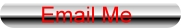HOME Electronics Directory Articles/ Tutorials eBooks About Us FORUM Links Contact Us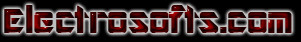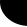Theory Of Semiconductors                                        by Gurudatha Pai

Einlitung: The world of electronics is developing faster & faster. The demand for quality software  is ever increasing, meanwhile the complexity of hardware is also on the rise. To understand how these diodes, transistors, thyristors, I.C.s & eventually the hardware itself work, it is necessary to understand the basic Physics behind these. That is noting but the "Theory Of Semiconductors ".

This article is a helping hand in understanding the basic concepts, which are dealt with in detail. A suitable analytical explanation is also provided for interesting readers who may be studying this subject as part of their course. But for those, who just want to have an overall idea, can simply skip the analytical description.

Prerequisites:   Let us list a few prerequisites,

i) An idea of atomic structure, Bohr's atomic model in particular.

ii) Crystal structure basics.

iii) An idea of Heisenberg's Uncertainty Principle.

iv) Wave Mechanics & Schrödinger Wave  Equation.

v) De Brooglie's wave Hypothesis of "Wave nature of matter".

Although all these are not necessary but their knowledge is an advantage. A  High School level of study of these concepts is more than enough for our discussion.

Now Let us move on to the real thing,

Let Us brief Ourselves With a little bit of preliminaries.

Atomic Structure:  After a series of theories for the structure of an atom the most successful were Rutherford's Model & Bohr's model.
We shall not see Rutherford's model, only a small account of Bohr's model of Hydrogen and Hydrogen like particles.

Bohr's model of Hydrogen Atom

Bohr's atomic model is a refined version of Rutherford's model. it assumes the following which

as we will see later, not to have any  logical or theoretical explanation then.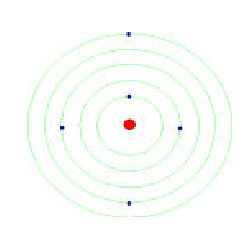Postulates of Bohr's Theory:-

i) Electrons revolve round the positively charged nucleus in certain definite circular orbits having certain definite energy.

ii) These are "stationary orbits " & Electrons can stay in these orbits without radiating energy.

iii) Electrons absorb or emit energy whenever they jump from one energy level to another in accordance with Planck's Quantization Rule.

i.e.                           ΔE=hγ                 where,   ΔE is the change in energy

h is the Planck's constant

γ is the frequency of the of light emitted.

iv) Electrons revolve in only those circular orbits, in which the angular momentum of the electron is Quantized (Quantization Rule).

i.e.                        mvrn =nh/(2Π)                    n= 1, 2, 3, . . . .

The graph energy vs. frequency is shown here: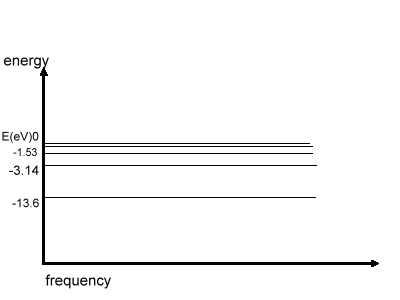The above Fig. shows the energy distribution in various levels in Hydrogen atom, E=0 when n= infinity, i.e. when e-  is out of the atom.

Note: We have not derived the values of energy, which can be done assuming the  Coulomb's Law & Bohr's Quantization Rule.
We may even derive the values of radius of Bohr's orbit & velocity of e-  in the orbit.*

Drawbacks of the Theory:- 1) It could not explain the fine structure of  spectrum.
2) No Explanation for the Quantization Rule.

3)Uncertainity Principle Completely eliminates the possibility of having e-s in specific circular orbits.
4)It cannot be applied to multi-electron systems.

Take Home: An atom consists of a positively charged nucleus around which e- revolve in definite quantized energy circular orbits of only certain radii.

So, we shall be done with Bohr's model & move on to a somewhat complicated Schrödinger Wave Equation Solutions of Hydrogen atom & further switch to, that our interest, Silicon atom.

*All the derivations are not done here, the reason being the irrelevancy of the same in our study.
This Discussion of Bohr's Model can be extended to single e- systems having any atomic number.

Before we move on Schrödinger wave equation solutions of Hydrogen atom, we shall learn something about  Heisenberg's uncertainty Principle.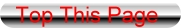Heisenberg's uncertainty Principle

Heisenberg's uncertainty Principle  state that,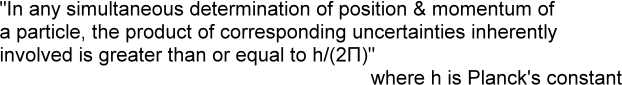i.e.

Δx. Δpx ≥ h/(2Π)
Even the following are true according to Heisenberg's uncertainty Principle,
ΔE . Δt ≥ h/(2Π),
Δθ . ΔL ≥ h/(2Π)            where,
Δx is the uncertainty in position
Δpx is the uncertainty in momentum
E is the energy of the particle, t is the time spent the particle in the energy state E,
θ is the angular position, L is the angular momentum
Δ represents the change or uncertainty

This means , we cannot measure the momentum & position of a particle accurately, all we can say is the probability of finding the particle in a region of space.

* The proof for  Heisenberg's uncertainty Principle is out of the purview of our domain.
Although  Heisenberg's uncertainty Principle is inherently applicable to  every system, it is not observable in macro scale .Schrödinger equation solutions of Hydrogen atom

Einlitung: Luis De-Brooglie Hypothecated that if light can behave as waves & particles, particles will also behave as waves under suitable conditions. Due to the inherent wave nature of particles it is not possible to pin point the location of a particle such as e-. We also know that, a wave in space is always descripted by a differential equation known as 'Wave equation' of the wave. Schrödinger came out with an interesting mathematical theory which gave the wave equation of matter.  Though, the  mathematical function in Complex no.s did not have a direct physical interpretation but later was found to give the probability of finding the particle if Squared & taken modulus of as defined in complex domain.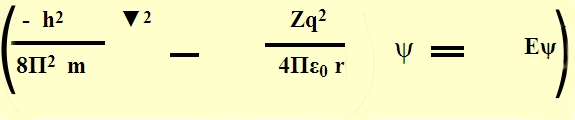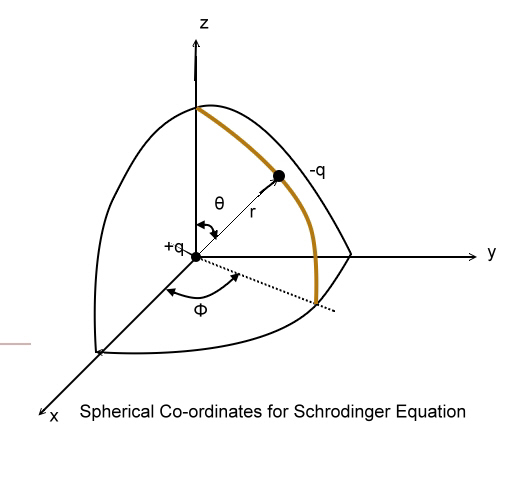We shall not say any further about the form of Schrödinger wave equation the mathematical conditions posed on it & so on.

Potential Energy Distribution in hydrogen Atom:
The Hydrogen atom can be thought  to have a nucleus of charge (+q)  = 1 C. fixed in position. The fig. shows the potential energy distribution of Hydrogen atom, in which on  e can observe that the potential energy (-ve) of the atom is highest at infinity distance from the centre & zero at nucleus. So, we have a complex Boundary conditions & a solution in 3-d.
We shall not solve the Schrödinger wave equation But assume the same & proceed.
The Schrödinger wave equation is solved in polar coordinates by putting the boundary conditions.
The solution  is of the form,
ψ (r,θ,Φ)=R(r).P(Φ).Q(θ)

When we solve assuming the above as solution, to obtain a physically feasible solutions , it requires us to have 3 Quantum no.s n, l, mc called the Principal, azimuthal and Magnetic Quantum no.s.

Principal Quantum Number (n) :-
It is a measure of total energy of the e- .
It is also a measure of major axis of the elliptical orbit.
The energy levels are indicated as K, L, M, N, ... shells rather than orbits. This is due to the inability to pin point the location of the e- as  per Heisenberg's uncertainty Principle.

That means, there is no concept of radius of orbit as predicted by Bohr, but all we can say is that, 'there is a high probability of locating an e- at the distance given by the Principal Quantum Number'.

Further, we have Azimuthal Quantum Number (l), Magnetic Quantum Number (ml) and Spin Quantum Number (ms) obtained while solving the
Schrödinger equation. Which give information about the Quantized Angular Momentum, Direction of Angular Momentum and spin on the e- respectively.

Observe The Table below and also the potential well of Hydrogen atom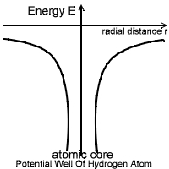Shell n>0 0≤ l ≥n-1 -l ≤ ml ≤ l ms = ± 1/2 Levels Max Number of  e- n l ml ms K 1 0 0 ± 1/2 1s 2 L 2 0 0 ± 1/2 2s 2 1 0 ± 1/2 2p 6 1 -1 ± 1/2 1 +1 ± 1/2 M 3 0≤ l ≥2 -2 ≤ ml ≤ 2 ± 1/2 3s 2 ± 1/2 3p 6 ± 1/2 3d 10

Table of Quantum Number

So, Now in the next article we will see what impact does this concept make on multi electron systems and Silicon in particular.About next Article: This was just preliminaries to semiconductor theory. In the coming articles, that will be available online soon, we will learn the atomic structure of the Silicon atom, Silicon Crystal, intrinsic and extrinsic Semiconductors and further.